# Class 6 Maths NCERT Solutions for Chapter 7 Fractions Ex – 7.3

## Fractions

Question 1.

Write the fractions. Are all these fractions equivalent?Solution: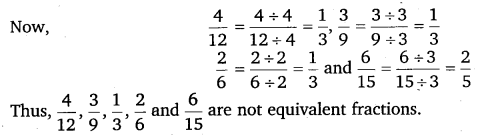Question 2.
Write the fractions and pair up the equivalent fractions from each row.Solution: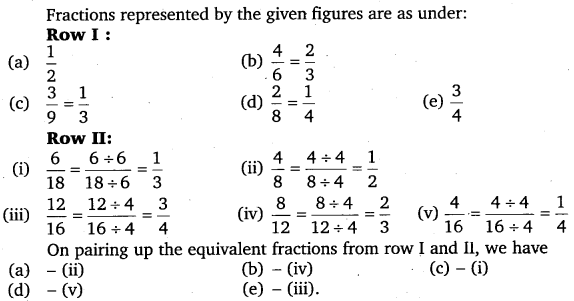Question 3.
Replace □ in each of the following by the correct number: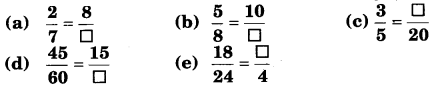Solution: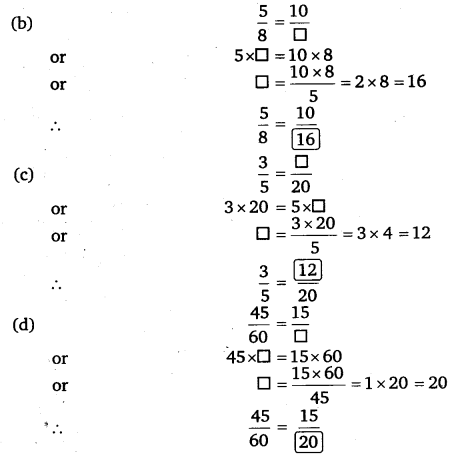Question 4.
Find the equivalent fraction of$\frac { 3 }{ 5 }$ having
(a)
denominator 20
(b) numerator 9
(c) denominator 30
(d) numerator 27

Solution:Question 5.
Find the equivalent fraction of$\frac { 36 }{ 48 }$ with

(a) numerator 9
(b) denominator 4

Solution:
(a)
∵ 36 ÷ 9 = 4
∴ We divide the numerator and denominator by 4$\frac { 36 }{ 48 } =\frac { 36\div 4 }{ 48\div 4 } =\frac { 9 }{ 12 }$
(b) ∵ 48 ÷ 4 = 12
∴ We divide the numerator and denominator by 12$\frac { 36 }{ 48 } =\frac { 36\div 12 }{ 48\div 12 } =\frac { 3 }{ 4 }$

Question 6.
Check whether the given fractions are equivalent: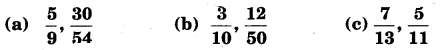Solution:Question 7.
Reduce the following fractions to simplest form: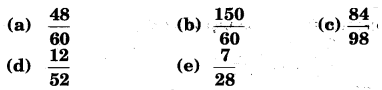Solution: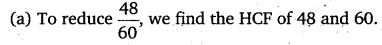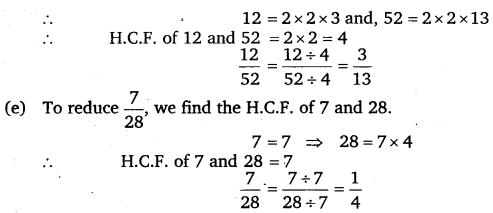Question 8.
Ramesh had 20 pencils, Sheelu had 50 pencils and Jamaal had 80 pencils. After 4 months, Ramesh used up 10 pencils, Sheelu used up 25 pencils and Jamaal used up 40 pencils. What fraction did each use up? Check if each has used up an equal fraction of their of her/his pencils?

Solution:
Fractions of pencils used by Ramesh, Sheelu and Jamaal are$\frac { 10 }{ 20 } ,\frac { 25 }{ 50 }$ and$\frac { 40 }{ 80 }$ respectively.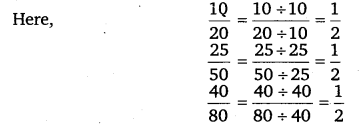Thus, these fractions are equal.

Question 9.
Match the equivalent fractions and write two more for each: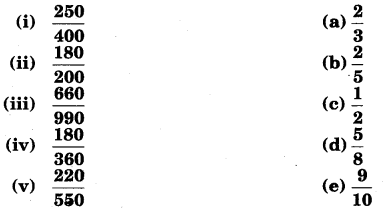Solution: